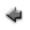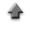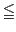previoushome

# 3 dimensional function model fitting

You can fit an arbitrary 3 dimensional function model v=f(x,y,z) to a data set including x, y, z, and v. To start, click "3D Fit" tab on the application.
If you use the sample data, click here, and save it in the appropriate directory (or folder).

When you fit a defalt function to the sample data,

1. Click "Open data file", and specify the data file prepared according to the above instruction.
2. When clicking the "Fit" button, a result of the fitting is displayed.

## Data

1. Each label for data can be set in the first row in the data file. The label can not include spaces. Each label has to be delimited by space or tab.
2. Data must be inputted by normal width.
3. Data must be inputted in the data file according to the data type of v.
• When no errors are included in v data or v is Poisson-type data, the data must be inputted in order of x, y, z and v which the values are delimited by space or tab. Change the line, and input the data of the following row similarly until the input of the last data ends.
 x1 y1 z1 v1 x2 y2 z2 v2 . . . . . . . . . . . . xn yn zn vn
• When v is Gaussian-type data and errors are included in v, the data must be inputted in order of x, y, z, v and the error of v which are delimited by space or tab. Change the line, and input the data of the following row similarly until the input of the last data ends.
 x1 y1 z1 v1 σv1 x2 y2 z2 v2 σv2 . . . . . . . . . . . . . . . xn yn zn vn σvn
4. In addition, you can use the table data form in the data file. You may include the column data in the data file which are not used to fit. In that case, the specification of the columns in the data file used to fit is needed at the line "Data columns" in the following "Input" instruction.

## Inputs

1. Click "Open data file", and specify the data file prepared according to the above instruction.
2. When the labels for data are used, check the "Data labels".
3. The columns in the data file to be used to fit a function model can be specified at the line "Data columns". For instance, in the case that the data type is "unknown errors", when x are put in the third column, y in the forth column, z in the fifth column and v in the sixth column in the data file, specify "3 4 5 6", and check the "Data columns" box.
4. The range for x data to be fitted can be specified at the line "x data range". In that case, specify the range for x axis (the numerical values must be delimited by space), and check the "x data range" box. For instance, when you want to assume the range for x to be 0x15, specify "0 15", and check the "x data range" box.
5. Like the range for x data, the range for y data to be fitted can be specified at the line "y data range". In that case, specify the range for y in the plot (the minimum and the maximum values must be delimited by space), and check the "y data range". For instance, when you want to assume the range for y in the histogram to be 0y15, specify "0 15", and check the "y data range".
6. The range for z data to be fitted can be specified at the line "z data range". In that case, specify the range for z in the plot (the minimum and the maximum values must be delimited by space), and check the "z data range". For instance, when you want to assume the range for z in the histogram to be 0z15, specify "0 15", and check the "z data range".
7. Select the data type at the line "Data type" according to the data type.
• When no errors are included in v data, select "unknown errors".
• When v is Gaussian-type data and errors are included in v data, select "Gaussian".
• When v is Poisson-type data, select "Poisson".
8. Input a function model to be fitted at the line "Function f(x,y,z)", and click the "Set" button. About the input of the function, refer here. The input of the function must follow the form v=f(x,y,z).
9. This fitting algorithm requires the specification of initial values of the parameters included in the function model. Then, you must input the initial values of the parameters at the line "Parameters" which must be delimited by space, and click the "Set" button.
10. A result of fitting can be displayed, when the "Fit" button is clicked, and the fitting function is also displayed on the graph.
11. When the "Show error matrix" button is clicked, the elements of the error matrix is displayed.
12. When the "Show residual graph" button is clicked, the plot of the residuals is displayed in another window.
13. When the "Show residuals" button is clicked, the residual table is displayed.

Kazushi Neichi